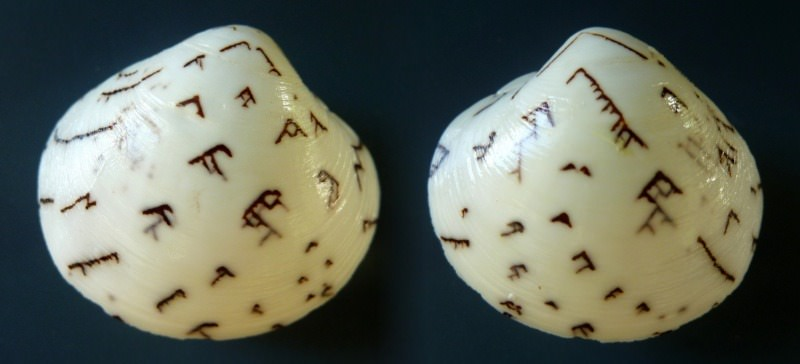# Sierpinski TriangleAnything that's covered here is almost definitely covered in a very well known webpage, The Sierspinski Triangle Page to End Most Sierspinski Triangle Pages but regardless, I must press on.

Let's draw a triangle.

Woah. Holy repetition! Repetition is the bane of the programmer's existence.

Let's use the repeat command to remove that repetition.

Neat. Now what is a Sierpinski triangle?

A Sierpinski triangle is the shape that emerges when we say: 'how does one draw a triangle? SIMPLE! One draws three smaller triangles!

A partial implementation of that is as follows.

Actually I told a fib. When I said: "A Sierpinski triangle arises when we say: 'how does one draw a triangle? SIMPLE! One draws three smaller triangles!' "

I should've said:

How does one draw a Sierpinski triangle? SIMPLE! One draws three smaller *Sierpinski* triangles!

But this is an example of unbounded recursion. We need to specify some kind of boundary condition, to stop the recursion before the computer sets on fire. (Or before the program fails with a stack overflow error. Whichever is first.)

Here's a pretty good boundary condition: What if they are too small? Then one simply draws a regular triangle.

What we need is a 'stack counter' as described in the Koch curve article. A way to say 'if the stack depth is greater than our limit, just draw a triangle, otherwise, draw a Sierpinski!'

That worked smashingly.

But it might be easier to see how it worked so smashingly if we look at the simplest version of the Sierpinski, followed by some more complex examples.

Another technique for drawing the Sierpinski triangle in logo is to use a curve, specifically, the Sierpinski Arrow-Head Curve, as shown below.

Technically this isn't a Sierpinski triangle. It's a curve that approximates a Sierpinski triangle. We go even further than that. We can say that at its limit it approaches a Sierpinski triangle. But that's hardly the same thing now is it?

Sierpinski, by the way, in case you were a little concerned, didn't just sit around all day thinking about triangles. In his time between thinking about triangles he managed to write some 50 books*, and he also created 'Sierpinski's Curve' which is a completely different thing and doesn't involve triangles. Okay, it is a little bit similar. He made a carpet too.

(*I like to imagine that all 50 books were just animated flip-books showing a deeper and deeper zoom into the Sierpinski triangle.)

What will he get up to next!